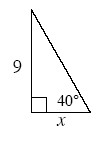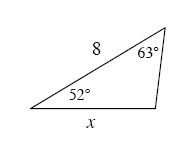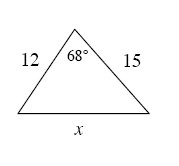### Home > GC > Chapter 6 > Lesson 6.2.5 > Problem6-83

6-83.

Examine the triangles below. For each, solve for $x$ and name which tool you use. Show all work.

1.What trig ratio can you use to solve for $x$?

$x\approx10.73$, tangent

1.Find the measure of the unknown angle. Now what law can you use to solve for $x$?

1.Use the Law of Cosines to solve for $x$.

$x\approx15.30$, Law of Cosines